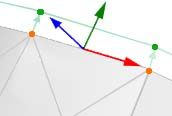# modify position of points on boundary of polydata surface

Hello, I am trying to modify the coordinates of points on the boundary of a triangulated surface (polydata made of triangle cells), in order to slightly expand some sections of the boundary in a direction that is perpendicular to local the boundary edge and parallel to the local boundary face. Basically in the green direction in this sketch.I figured out I need to:

1. Select the boundary edges.

2. For each edge, select the two orange points and the triangle.

3. From the triangle, get the normal (the blue vector in my sketch).

4. From the edge, get the red vector.

5. With a scalar product between blue and red, get the green vector.

6. Check the correct direction of the green vector pointing outwards (I guess I can do this comparing the green vector with one vector obtained joining the center of the triangle with the center of the edge).

7. Move the coordinates of the points along a vector parallel to green and with a specified length.

(*) If I need to move just some section of the boundary, I guess I can “mark” the nodes to be moved with a scalar property.

This should do the trick, but I have the following problems.

(A) I can find all the boundary edges with vtkFeatureEdges() (with only BoundaryEdgesOn()), but as I understand it, this filter outputs a copy of the edges, not a reference to the original edges that is what I need.

(B) If I can select an edge, how can I select the points and the triangle?

Then I can easily get the coordinates and the normal, but I need help to solve (A) and (B).

Thanks very much!

Hello again! I have found some possible solutions.

(1) It looks like that using the function described in topic 2530 it would be possible to extract an array of all the ids of the boundary nodes. In this case, I am still looking for a method to get the cell (triangles) ids and the edge ids (boundary edge of these triangles).

(2) Using vtkGeometryFilter() with options PassThroughCellIdsOn() and PassThroughPointIdsOn() might do the trick. However I am still looking for examples on how to use this to select boundary edges (topological dimension 1) of a triangulated surface (made of triangle cells). Actually, it is not clear to me what the input and output of vtkGeometryFilter() is, and what options I have to get what I need: points, edges and triangles along the boundary of a surface, all correlated (e.g. triangle A has boundary edge B with nodes C and D).

Thanks!